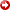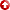--> --> Best Fitting of NMO Equations and Depth Conversion
[First Hit]# Best Fitting ofNMOEquations and Depth Conversion

## Abstract

The hyperbolicNMOequation is only exact for one interface in homogeneous and isotropic media. In heterogeneous media with many interfaces, this equation is an approximation and does not perform well at large offsets. To solve this problem, we consider several non-hyperbolicNMOequations, based on the average, RMS and root-mean-quartic (RMQ) velocities. Moreover, we explore the possibility of using alternative approaches, other than the standard Dix equation based on the root-mean-square (RMS) velocities, to obtain the interval velocities and perform the depth conversion to locate the interfaces. We consider two velocity models to test theNMOequations, compute a common midpoint (CMP) synthetic seismogram and perform a non-linear inversion to obtain the traveltimes. One of the models has a velocity inversion, which poses a challenge to the approximations. We find that the performance of the approximations is model dependent, so a priori information of the velocity profile can be useful to perform a suitable inversion, or an optimal stack of the reflection events is required to test theNMOcorrection. Moreover, the results show that the inversion based on the RMS velocities yields better results than those based on the RMQ velocities, but this is not always the case. On the other hand, the inversion using the average velocity performs a worse velocity depth estimation, even compared to the RMS results obtained with the hyperbolic approximation.